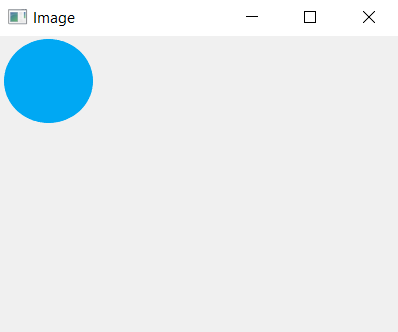Open in App
Not now

# PyQt5 – How to add image in window ?

• Last Updated : 03 Aug, 2021

In this article, we will see how to add image to a window. The basic idea of doing this is first of all loading the image using QPixmap and adding the loaded image to the Label then resizing the label according to the dimensions of the image, although the resizing part is optional.

In order to use Qpixmap and other stuff we have to import following libraries:

```from PyQt5.QtWidgets import *
from PyQt5.QtGui import QPixmap
import sys```

Syntax :

`pixmap = QPixmap('image.png')`

Argument : Image name if image is in same folder else file path.

For adding image to label :
Syntax :

`label.setPixmap(pixmap)`

Argument : It takes QPixmap object as argument.

Code :

## Python3

 `# importing the required libraries` `from` `PyQt5.QtWidgets ``import` `*``from` `PyQt5.QtGui ``import` `QPixmap``import` `sys`  `class` `Window(QMainWindow):``    ``def` `__init__(``self``):``        ``super``().__init__()` `        ``self``.acceptDrops()``        ``# set the title``        ``self``.setWindowTitle(``"Image"``)` `        ``# setting  the geometry of window``        ``self``.setGeometry(``0``, ``0``, ``400``, ``300``)` `        ``# creating label``        ``self``.label ``=` `QLabel(``self``)``        ` `        ``# loading image``        ``self``.pixmap ``=` `QPixmap(``'image.png'``)` `        ``# adding image to label``        ``self``.label.setPixmap(``self``.pixmap)` `        ``# Optional, resize label to image size``        ``self``.label.resize(``self``.pixmap.width(),``                          ``self``.pixmap.height())` `        ``# show all the widgets``        ``self``.show()` `# create pyqt5 app``App ``=` `QApplication(sys.argv)` `# create the instance of our Window``window ``=` `Window()` `# start the app``sys.exit(App.``exec``())`

Output :My Personal Notes arrow_drop_up